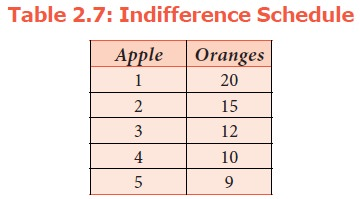Home | | Economics 11th std | Ordinal Analysis (or) Ordinal Utility Approach (or) Hicks and Allen Approach (or) Indifference Curve Analysis

# Ordinal Analysis (or) Ordinal Utility Approach (or) Hicks and Allen Approach (or) Indifference Curve Analysis

Ordinal Analysis (or) Ordinal Utility Approach (or) Hicks and Allen Approach (or) Indifference Curve Analysis

Ordinal Analysis (or) Ordinal Utility Approach (or) Hicks and Allen Approach (or) Indifference Curve Analysis

## Introduction

F.W.EdgeWorth (English Economist) and Vilfredo Pareto (Italian Economist) criticised the Cardinal Utility Approach. They assumed that utility cannot be measured absolutely, but can be compared or ranked or ordered by ordinal numbers such as I, II, III and so on. Edgeworth first developed a more scientific approach to the study of consumer behaviour, known as “Indifference Curve Approach” in1881. In 1906, Vilfredo Pareto modified the “Edgeworth Approach”. Again J.R.Hicks and R.G.D.Allen refined the Indifference Curve Approach in 1934. Later, in 1939 J.R.Hicks in his book “ Value and Capital” gave a final shape to this “Indifference Curve Analysis”.The theory of indifference curve was given by J R Hicks and RJD Allen, ‘A reconsideration of the theory of value’, Economics in 1934.

## Scale of Preference

This theory is also based on scale of preference. A rational consumer usually prefers the combination of goods which gives him maximum level of satisfaction. Thus, the consumer can arrange goods and their combination in order of their satisfaction. Such an arrangement of combination of goods in the order of level of satisfaction is called the “Scale of Preference”.

## Assumptions

1.        The consumer is rational and his aim is to derive maximum satisfaction.

2.        Utility cannot be cardinally measured, but can be ranked or compared or ordered by ordinal number such as I, II, III and so on.

3.        The Indifference Curve Approach is based on the concept “Diminishing Marginal Rate of Substitution”.

4.        The consumer is consistent. This assumption is called as the assumption of transitivity. If the consumer prefers combination A to B and B to C, then he should prefer A to C. If A>B and B>C, then A>C.

## An Indifference Schedule

An indifference schedule may be defined as a schedule of various combinations of two commodities which will give the same level of satisfaction. In other words, indifference Schedule is a table which shows the different combination of two goods that gives equal satisfaction to the consumer.Table has five combinations of two commodities Apple and Orange. Each of these combinations give the consumer the same level of satisfaction without discrimination. In the schedule, the combinations are arranged in such a way that the consumer is indifferent among the combinations. Hence, this schedule is called as, “Indifference Schedule”. He will neither be better off nor worse off whichever combination he chooses.

Tags : Economics , 11th Economics : Chapter 2 : Consumption Analysis
Study Material, Lecturing Notes, Assignment, Reference, Wiki description explanation, brief detail
11th Economics : Chapter 2 : Consumption Analysis : Ordinal Analysis (or) Ordinal Utility Approach (or) Hicks and Allen Approach (or) Indifference Curve Analysis | Economics